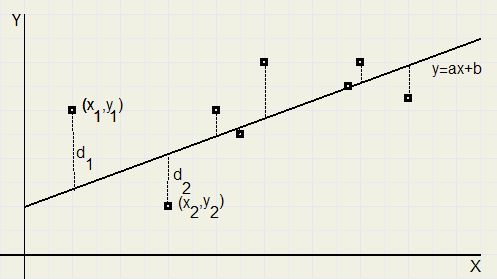The Least Squares methodIntroduction
The relation between two numbers may be defined by
• a table
• a coordinates system
• an equationEach (x,y) pair we may paint as a point in a coordinate system.
Then we may search for the best fitting polynomial.

Note: a polynomial degree n is: ......y = c0+c1x+c2x2+........+cnxn

please look at the figure above:

painted are points (x1,y1)................and asked is the best fitting polynomial degree 1 through these points.

"Best fitting" means, that the sum of the squared differences for each point is minimal.
These differences are painted as dotted lines in the figure.

The applied "Least Squares" method to find the best fitting polynomial is a nice application of linear algebra.

My equation grapher Graphics-Explorer uses this method,
the degree may be 0 to 7.

The Least Squares method
Given are points (x1,y1) , (x2,y2)...(xn , yn)

requested:
a polynomial degree m, y = c0 + c1x + c2x2 + ... + cmxm through these points having the minimal deviation.

If all points are exactly on the the polynomial, so m+1 = n, we have:

y1 = c0 + c1x1 + c2x12 + ... + cmx1m
y2 = c0 + c1x2 + c2x22 + ... + cmx2m
.............
.............
yn = c0 + c1xn + c2xn2 + ... + cmxnm
written in matrix form:

 ­ y1 ­ y2 ... ... yn
=  ­ 1 x1 1 x1 2 ... x1 m ­ 1 x2 1 x2 2 ... x2 m .. .. .. .. .. .. .. .. .. .. 1 xn 1 xn 2 ... xn m
 ­ c0 ­ c1 .. .. cm

or shorter: y = M . c
If the points are not exactly on the polynomial, there will be a difference vector:

y - M . c

The norm of this difference vector is the sum of the squared differences.

So, we look for c , where || y - M . c || is minimal.

this will be the case if the difference vector is perpendicular to the column space of M.
The inner product is zero in this case.

(M c )t ( y - M c) = 0

(ct Mt) (y - M c) = 0

ct ( Mt ( y - M c)) = 0

ct ( Mt y - Mt M c ) = 0

so:
Mt y - Mt M c = 0

Mt M c = Mt y

(Mt M)-1Mt M c = (Mt M)-1Mt y

c = (Mt M)-1 Mt y
remarks
1.
Mt means matrix M transposed, mirrored in it's diagonal, so writing the rows as columns.

if
M =  ­ 2 0 3 ­ 1 5 8
then

M t

=
 ­ 2 1 ­ 0 5 3 8
2.
rule: ( A B)t = BtAt

3.
The inner product of two vectors a en b may be written as at.b

4.
In the case of linear regression, where m = 1 and c =[b,a] .......{because the line has the equation y = b + ax..}
this is true:

y

=

 ­ y1 ­ y2 ... yn

M

=

 ­ 1 x1 ­ 1 x2 ... ... 1 xn

Mt =  ­ 1 1 ... 1 ­ x1 x2 ... xn
starting with
MtMc = Mty

 ­ 1 1 ... 1 ­ x1 x2 ... xn

·

 ­ 1 x1 ­ 1 x2 ... ... 1 xn

 ­ b ­ a

=

 ­ 1 1 ... 1 ­ x1 x2 ... xn

·

 ­ y1 ­ y2 ... yn

­n Σ xi
­
 Σ xi
 Σ xi 2
·  ­ b ­ a
=
­ Σ yi
­
 Σ xi yi

­b n + a  Σ xi
­
b  Σ xi
+ a  Σ xi 2
=
­ Σ yi
­
 Σ xi yi
The next system of linar equations has to be solved:
b n + a  Σ xi
=  Σ yi

b  Σ xi
+ a  Σ xi 2
=  Σ xi yi

or
 Σ xi yi
− a  Σ xi 2
− b  Σ xi
= 0

 Σ yi
− a  Σ xi
− b n = 0
of
 Σ (xi yi − a xi 2 − b xi)
= 0

 Σ (yi − a xi − b)
= 0
For the solution please refer to my article Linear Regression
See formula's ....1) and ...........2)

Example
Find the least square straight line through points.........(0,1) (1,3) (2,4) en (3,4)

M

=

 ­ 1 0 ­ 1 1 1 2 1 3

M t =  ­ 1 1 1 1 ­ 0 1 2 3

M t M =  ­ 4 6 ­ 6 14

(M t M) −1 = 0 , 1  ­ 7 −3 ­ −3 2

c = 0 ,

1  ­ 7 −3 ­ −3 2
 ­ 1 1 1 1 ­ 0 1 2 3

 ­ 1 ­ 3 4 4

=

 ­ 1.5 ­ 1

So, the line is y = 1.5 + x